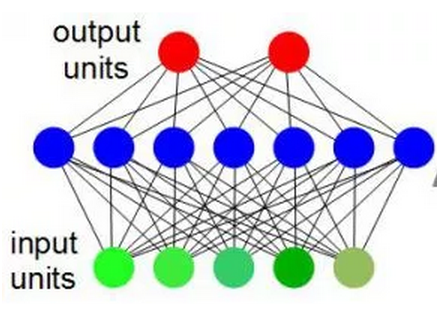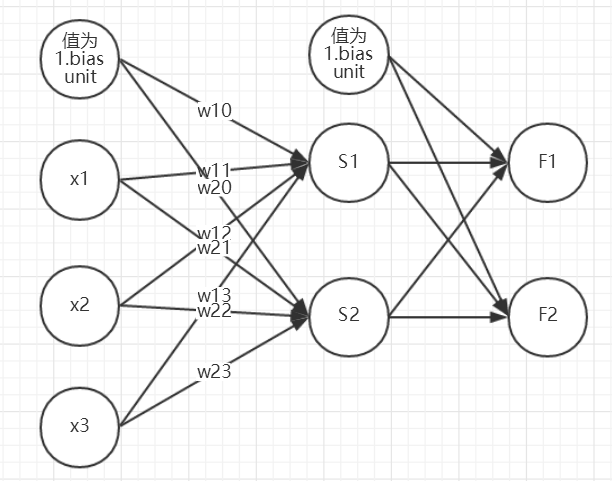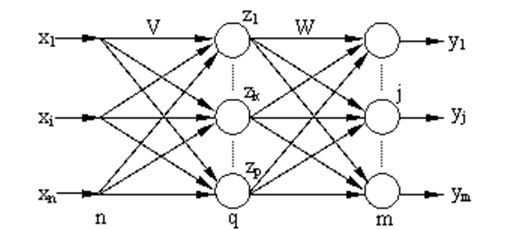# 机器学习基础——BP算法

## BP后向传播算法

### 一、BP神经网络的基本原理

BP（Back Propagation）网络是1986年由Rinehart和McClelland为首的科学家小组提出，是一种按误差逆传播算法训练的多层前馈网络，是目前应用最广泛的神经网络模型之一。BP网络能学习和存贮大量的输入-输出模式映射关系，而无需事前揭示描述这种映射关系的数学方程。它的学习规则是使用最速下降法(梯度下降法)，通过反向传播来不断调整网络的权值和阈值，使网络的误差平方和最小。BP神经网络模型拓扑结构包括输入层（input）、隐层(hide layer)和输出层(output layer)（如下图所示）。上图中，绿色单元为输入单元(input unit)，蓝色单元为隐藏层单元(hidden unit)，红色单元为输出单元(output unit)。


### 二、BP神经元与前向传播算法简介

• 神经元
假设我们的训练集只有一个实例x(1),y(1)$（x(1),y(1)）$，我们的神经网络是一个三层的神经网络，即隐藏层只有1层。以中间层神经元Sj$S_j$，(j=1,2)为例，它只模仿了生物神经元所具有的三个最基本也是最重要的功能：加权、求和与转移。其中x1x2x3$x_1、x_2、x_3$分别代表来自输入层(Input Layer)神经元1、2、3的输入；wj1wj2wj3$w_{j1}、w_{j2}、w_{j3}$则分别表示神经元1、2、3与第j个神经元的连接强度，即权值；wj0$w_{j0}$为阈值f()$f(·)$为传递函数；yj为第j个神经元的输出。
第1个神经元的净输入值 S1$S_1$为：
Sj=i=13wjixi+wj0=WjX

其中，wj0$w_{j0}$ 是偏置单元x0$x_0$对应的权值，x0$x_0$为1。
用上图的S1$S_1$ 为例，S1=w11x1+w12x2+w13x3+w10x0$S_1 = w_{11}x_1+w_{12}x_2+w_{13}x_3+w_{10}x_0$

yj=f(Sj)=f(i=03wjixi)

• 前向传播算法步骤
a(1)=x

z(2)=θ(1)a(1)

a(2)=f(θ(1)a(1))(bias unit)

z(3)=θ(2)a(2)

a(3)=Fθ(x)=f(θ(2)a(2))(bias unit)

a(3)$a^{(3)}$ 为预测结果。

### 三、反向传播#### 3.1 定义误差函数

Ep=12j=1m(tpjypj)2

E=12p=1Pj=1m(tpjypj)2

#### 3.2 输出层权值的变化

Δwjk=αEwjk=αwjkp=1PEp

δyj=EpSj=EpyjyjSj

Sj$S_{j}$ 为前向传播中的z(3)$z^{(3)}$ 的第j个元素。yj$y_j$ 为前向传播中a(3)$a^{(3)}$ 的第j个元素

Epyj=yj[12j=1m(tpjypj)2]=(tpjypj)

yjSj=f2(Sj)

δyj=(tpjypj)f2(Sj)

Epwjk=EpSjSjwjk=δyjzk

Δwjk=p=1Pα(tpjypj)f2(Sj)zk

#### 3.3 隐藏层权值的变化

Δvki=αEvki=αvkip=1PEp=p=1P(αEpvki)

δzk=EpSk=EpzkzkSk

Sk$S_{k}$ 为前向传播中的z(2)$z^{(2)}$ 的第k个元素。zk$z_k$ 为前向传播中a(2)$a^{(2)}$ 的第k个元素。

Epzk=zk[12j=1m(tpjypj)2]=j=1m(tpjypj)yjzk

yjzk=yjSjSjzk=f2(Sj)wjk

zkSk=f1(Sk)

Epvki=EpSkSkvki=δzkxi

Δvki=Epvki=p=1Pj=1mα(tpjypj)f2(Sj)wjkf1(Sk)xi

### 四、BP算法的改进

BP算法理论具有依据可靠、推导过程严谨、精度较高、通用性较好等优点，但标准BP算法存在以下缺点：收敛速度缓慢；容易陷入局部极小值；难以确定隐层数和隐层节点个数。在实际应用中，BP算法很难胜任，因此出现了很多改进算法。
1） 利用动量法改进BP算法

ΔW(n)=αE(n)+βΔW(n1)

2） 自适应调整学习速率

3） 动量-自适应学习速率调整算法

4） L-M学习规则
L-M（Levenberg-Marquardt）算法比前述几种使用梯度下降法的BP算法要快得多，但对于复杂问题，这种方法需要相当大的存储空间。L-M(Levenberg-Marquardt)优化方法的权值调整率选为:

Δw=(JTJ+μl)1JTe

ps: